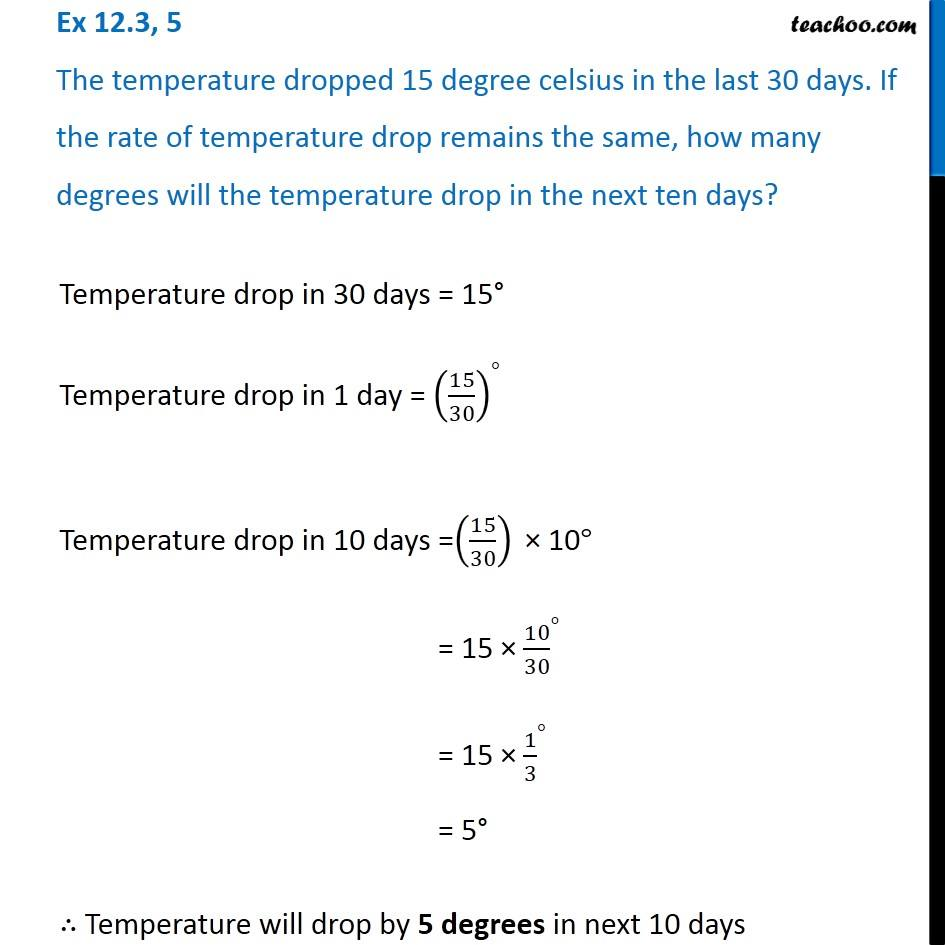1. Chapter 12 Class 6 Ratio And Proportion
2. Serial order wise
3. Ex 12.3

Transcript

Ex 12.3, 5 The temperature dropped 15 degree celsius in the last 30 days. If the rate of temperature drop remains the same, how many degrees will the temperature drop in the next ten days?Temperature drop in 30 days = 15° Temperature drop in 1 day = (15/30)^° Temperature drop in 10 days =(15/30)" × 10" ° = 15 × 〖10/30〗^° = 15 × 〖1/3〗^° = 5° ∴ Temperature will drop by 5 degrees in next 10 days

Ex 12.3

Chapter 12 Class 6 Ratio And Proportion
Serial order wise